# Residue Theorem¶

The Residue Theorem says that a contour integral of an analytic function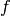over a closed curve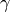(loop) is equal to the sum of residues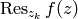of the function at all singularities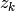inside the loop: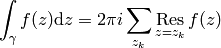Residue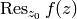is defined as the contour integral around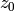divided by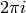: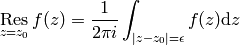and it is equal to the coefficient of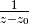in the Laurent series of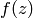around the point, as can be easily calculated: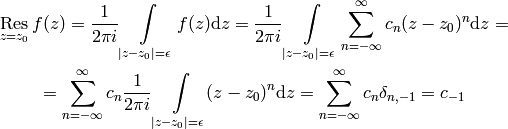where we used the result of the following integral (we integrate over the curve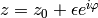,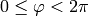, so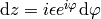):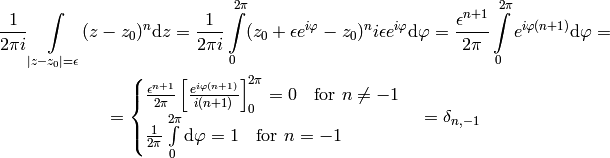## Computation of Residues¶

One has to calculate the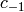coefficient in the Laurent series. One way to do that is to writeas: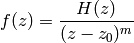where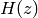is analytic in the vicinity of, e.g.has a pole of order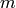at. Then: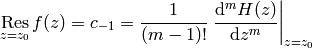in particular for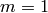: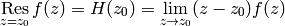for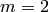:has a pole of order 1 at,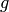is analytic at: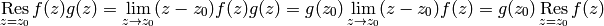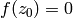, but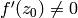andis analytic at: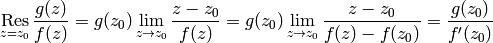## Useful Formulas¶

### Jordan’s Lemma¶

For estimating integrals over semicircles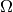(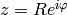,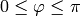), we can use the following estimates: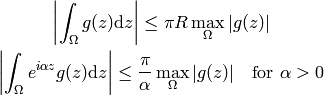(In the first case the integration path can be extended to the full circle if needed (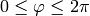), in the second case the semicircle is the maximum path. Also if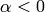, we need to integrate over the lower semicircle.) These formulas can be used to make sure the integral over the semicircle goes to zero as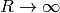. Intuitively speaking, in the first case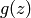must vanish faster than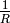(e.g.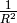is ok), in the second case it’s enough ifjust goes to 0 (no matter how fast).

The estimates can be proved easily: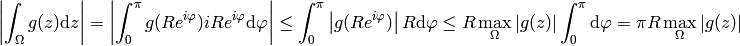and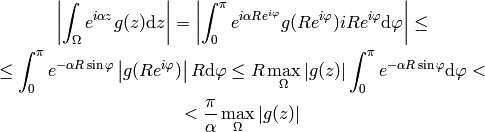where we use the following useful estimate for the integral (valid for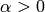):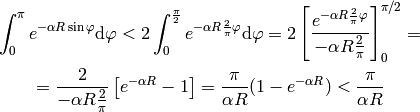### Other¶

Sometimes it is useful to integrate over the arc,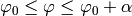, and let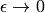at the end. If the function is analytic, the result is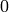. If the function has a pole of order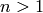, the result is infinity, unless it’s a full circle (in which case the result is 0). The remaining case is if the function has a pole of order one, e.g. it can be written (is analytic at):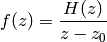Then: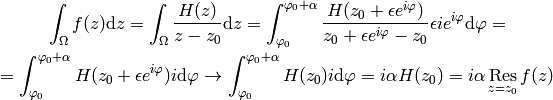## Complex Substitution¶

When substituting in integrals, as long as we just substitute for real functions, we use the regular substitution theorem, e.g.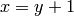(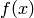can be a complex function):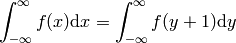if, on the other hand, we substitute for complex functions, e.g.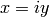: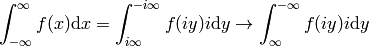then the first two integrals in the left hand side are equal, however the integral on the right hand side is over a different integration path and we need to use the Residue Theorem to relate those integrals, e.g. in general the two integrals on the LHS and the integral on the RHS are not equal. However the idea is that the integral after the substitution (and changing the limits, e.g. the integration path) is easier to evaluate, so the substitution guides us which integration path to choose for the Residue Theorem.